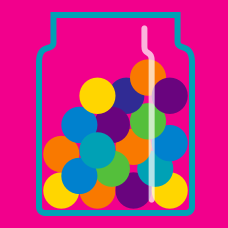Probability

# Discrete Conditional Probabilities

You throw two dice at the same time. The first die shows $5,$ and we don't know what the other die shows. Then what is the probability that the sum of outcomes of the two dice is $7?$

If two exclusive events $A$ and $B$ satisfy

$P\left(A \cap B^C\right) = \frac15, \quad P\left(A^C \cap B\right) = \frac14,$

what is the value of $P(A \cup B)$?

There are two identical boxes $A$ and $B.$ In box $A,$ there are two red balls and one white ball. In box $B,$ there are three red balls and two white balls. If you randomly chose one box and drew a white ball out of it, what is the probability that you chose box $A?$

When you roll two fair dice and the product $p$ of the values is four, what is the conditional probability distribution for the value $X$ of the first dice? Represent it in the form of $(P(X=1|p=4),P(X=2|p=4),\dots,P(X=6|p=4)) .$

Justin is taking out balls one by one from a box which contains $6$ red balls and $5$ blue balls. After he takes out $3$ balls from the box, he checks their colors: $2$ red and $1$ blue. What is the probability that the first ball he took out was red?

×